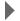Revision history [back]
There IS a relationship between T1 and correlation time which in turn is dependent on MW and viscosity. The relationship between rotational correlation time and T1 is given by the Bloembergen, Purcell and Pound equation (BPP) see (http://en.citizendium.org/wiki/Nmr_spectroscopy#NMR_spectroscopy:_Observable_parameters). The correlation time can be estimated from knowledge of MW and viscosity as follows: assuming a spherical shape the radius of hydration rh = ((MW)^0.44)/1.5 Use this radius to calculate volume of hydration Vh. According to the stokes-einstein relation the rotational diffusion coefficient Dr = kT/(6Vh*viscosity) Since rotational correlation time = 1/(6Dr) rotational correlation time = Vh*viscosity/(kT) k=Boltzmann constant and T=Temperature References: 1. Cantor and Schimmel : Biophysical Chemistry 2. M.Goldman : Quantum description of high-resolution NMR in liquids.

There IS a relationship between T1 and correlation time which in turn is dependent on MW and viscosity. However, this method will only given you a rough estimate.

The relationship between rotational correlation time and T1 is given by the Bloembergen, Purcell and Pound equation (BPP) see (http://en.citizendium.org/wiki/Nmrspectroscopy#NMRspectroscopy:Observableparameters).

The correlation time can be estimated from knowledge of MW and viscosity as follows:

assuming a spherical shape the radius of hydration rh = ((MW)^0.44)/1.5

Use this radius to calculate volume of hydration Vh.

According to the stokes-einstein relation the rotational diffusion coefficient Dr = kT/(6Vh*viscosity)

Since rotational correlation time = 1/(6Dr)

rotational correlation time = Vh*viscosity/(kT)

k=Boltzmann constant and T=Temperature

References:

1. Cantor and Schimmel : Biophysical Chemistry

2. M.Goldman : Quantum description of high-resolution NMR in liquids.

There IS a relationship between T1 and correlation time which in turn is dependent on MW and viscosity. However, this method will only given you a rough estimate.estimate. Also, I am not aware of any lab that actually uses this relationship. However, FYI:

The relationship between rotational correlation time and T1 is given by the Bloembergen, Purcell and Pound equation (BPP) see (http://en.citizendium.org/wiki/Nmrspectroscopy#NMRspectroscopy:Observableparameters).

The correlation time can be estimated from knowledge of MW and viscosity as follows:

assuming a spherical shape the radius of hydration rh = ((MW)^0.44)/1.5

Use this radius to calculate volume of hydration Vh.

According to the stokes-einstein relation the rotational diffusion coefficient Dr = kT/(6Vh*viscosity)

Since rotational correlation time = 1/(6Dr)

rotational correlation time = Vh*viscosity/(kT)

k=Boltzmann constant and T=Temperature

References:

1. Cantor and Schimmel : Biophysical Chemistry

2. M.Goldman : Quantum description of high-resolution NMR in liquids.

There IS a relationship between T1 and correlation time which in turn is dependent on MW and viscosity. However, this method will only given you a rough estimate. Also, I am not aware of any lab that actually uses this relationship. However, FYI:

The relationship between rotational correlation time and T1 is given by the Bloembergen, Purcell and Pound equation (BPP) see (http://en.citizendium.org/wiki/Nmr(relaxation time section in

http://en.citizendium.org/wiki/Nmrspectroscopy#NMRspectroscopy:Observableparameters). parameters ).

The correlation time can be estimated from knowledge of MW and viscosity as follows:

assuming a spherical shape the radius of hydration rh = ((MW)^0.44)/1.5

Use this radius to calculate volume of hydration Vh.

According to the stokes-einstein relation the rotational diffusion coefficient Dr = kT/(6Vh*viscosity)

Since rotational correlation time = 1/(6Dr)

rotational correlation time = Vh*viscosity/(kT)

k=Boltzmann constant and T=Temperature

References:

1. Cantor and Schimmel : Biophysical Chemistry

2. M.Goldman : Quantum description of high-resolution NMR in liquids.

There IS a relationship between T1 and correlation time which in turn is dependent on MW and viscosity. However, this method will only given you You may obtain a rough estimate. Also, I am not aware of any lab that actually uses this relationship. However, FYI:estimate of T1 using the following relationships.

The relationship between rotational correlation time and T1 is given by the Bloembergen, Purcell and Pound equation (BPP) (BPP equation) see (relaxation time section in

http://en.citizendium.org/wiki/Nmrspectroscopy#NMRspectroscopy:Observableparameters ).

The correlation time can be estimated from knowledge of MW and viscosity as follows:

assuming Assuming a spherical shape shape, the radius of hydration rh = ((MW)^0.44)/1.5

Use this radius radius, rh, to calculate volume of hydration hydration, Vh.

According to the stokes-einstein relation Stokes-Einstein relation, the rotational diffusion coefficient Dr = kT/(6Vh*viscosity)

Since kT/(6Vh*viscosity)

And Rotational correlation time = 1/(6*Dr)

Therefore, rotational correlation time = 1/(6Dr)

rotational correlation time = Vh*viscosity/(kT)Vhviscosity/(kT)

k=Boltzmann constant and T=Temperature

References:

1. Cantor and Schimmel : Biophysical Chemistry

2. M.Goldman : Quantum description of high-resolution NMR in liquids.6 No.5 Revision posted Feb 12 '10 at 12:32Evgeny Fadeev 577●1

There IS a relationship between T1 `T1` and correlation time which in turn is dependent on MW `MW` and viscosity. You may obtain a rough estimate of T1 `T1` using the following relationships.

The relationship between rotational correlation time and T1 `T1` is given by the Bloembergen, Purcell and Pound equation (BPP equation) see (relaxation time section in

http://en.citizendium.org/wiki/Nmrspectroscopy#NMRspectroscopy:Observableparameters `http://en.citizendium.org/wiki/Nmr_spectroscopy#NMR_spectroscopy:_Observable_parameters` ).

The correlation time can be estimated from knowledge of MW and viscosity as follows:

Assuming a spherical shape, the radius of hydration `rh = ((MW)^0.44)/1.5`

Use this radius, rh, `rh`, to calculate volume of hydration, Vh.`Vh`.

According to the Stokes-Einstein relation, the rotational diffusion coefficient `Dr = kT/(6Vh*viscosity)k*T/(6*Vh*viscosity)`

And Rotational correlation time `tau_r ``= 1/(6*Dr)`

Therefore, rotational correlation time = Vhviscosity/(kT)

k=Boltzmann `tau_r = Vh*viscosity/(k*T)`

`k=Boltzmann` constant and `T=Temperature`

References:

1. Cantor and Schimmel : Biophysical Chemistry

2. M.Goldman : Quantum description of high-resolution NMR in liquids.